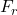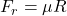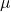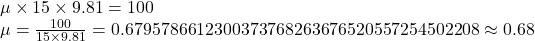## Devon is applying 100N of force to pull a 15 kg object, but the object isn’t moving. What does this indicate about the coefficient of

Question

Devon is applying 100N of force to pull a 15 kg object, but the object isn’t moving. What does this indicate about the coefficient of
friction for the surface?

in progress 0
2 months 2021-08-15T05:18:51+00:00 1 Answers 0 views 0

The maximum possible friction,is given bywhereis coefficient of friction and R=mg where m is mass and g is acceleration due to gravity. For the object to move, the applied force must be greater than the frictional force hence for this case